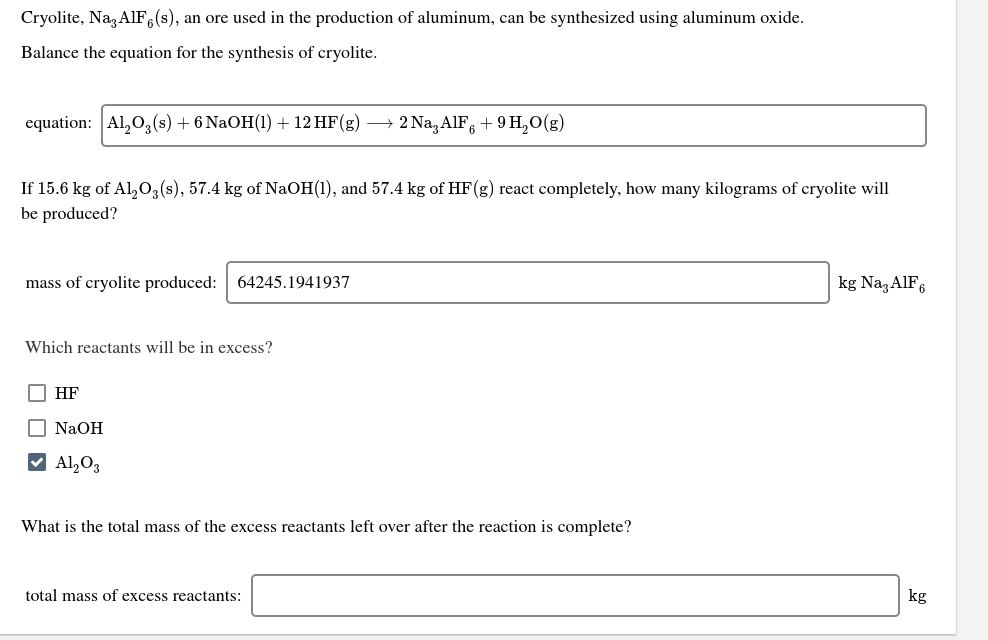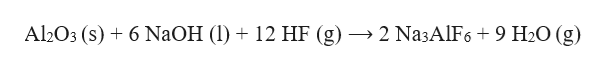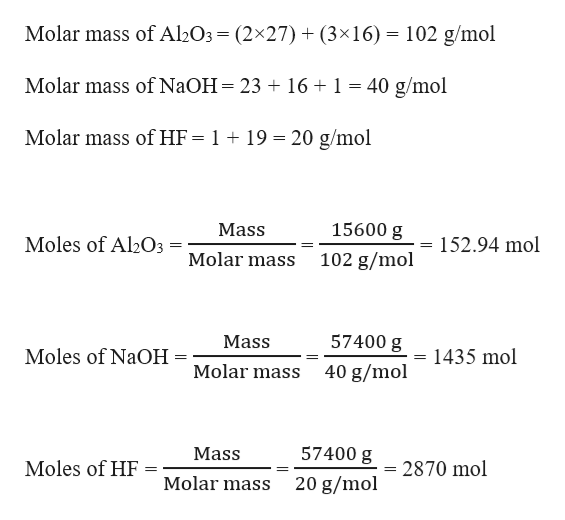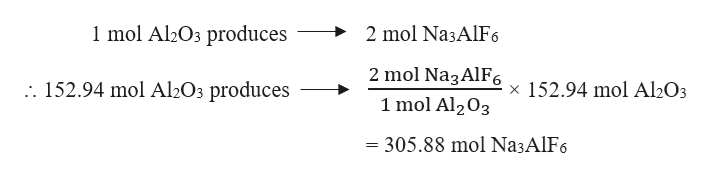# Cryolite, Na AlF,(s), an ore used in the production of aluminum, can be synthesized using aluminum oxide.Balance the equation for the synthesis of cryolite.2 Na, AIF, + 9 H,O(g)equation: Al, O,(s) + 6 NaOH(1) + 12 HF(g)If 15.6 kg of Al,03(s), 57.4 kg of NaOH(1), and 57.4 kg of HF(g) react completely, how many kilograms of cryolite willbe produced?kg Na,AIF6mass of cryolite produced:64245.1941937Which reactants will be in excess?O HFO NaOHV Al,O3What is the total mass of the excess reactants left over after the reaction is complete?kgtotal mass of excess reactants:

Question
17 viewshelp_outlineImage TranscriptioncloseCryolite, Na AlF,(s), an ore used in the production of aluminum, can be synthesized using aluminum oxide. Balance the equation for the synthesis of cryolite. 2 Na, AIF, + 9 H,O(g) equation: Al, O,(s) + 6 NaOH(1) + 12 HF(g) If 15.6 kg of Al,03(s), 57.4 kg of NaOH(1), and 57.4 kg of HF(g) react completely, how many kilograms of cryolite will be produced? kg Na,AIF6 mass of cryolite produced: 64245.1941937 Which reactants will be in excess? O HF O NaOH V Al,O3 What is the total mass of the excess reactants left over after the reaction is complete? kg total mass of excess reactants: fullscreen
check_circle

Step 1

A balanced chemical equation or reaction is the one in which the total number of atoms for each element in the reactant side (left hand side) is equal to the total number of atoms for each element in the product side (right hand side).

The balanced equation for the synthesis of cryolite can be written as:help_outlineImage TranscriptioncloseAl2O3 (s) + 6 NAOH (1) + 12 HF (g) → 2 Na3AlF6 + 9 H2O (g) fullscreen
Step 2

Given,

Mass of Al2O3 = 15.6 kg = 15600 g     (1 kg = 1000 g)

Mass of NaOH = 57.4 kg = 57400 g

Mass of HF = 57.4 kg = 57400 g

Moles of Al2O3 , NaOH and HF can be calculated as:help_outlineImage TranscriptioncloseMolar mass of Al2O3= (2×27) + (3×16) = 102 g/mol Molar mass of NaOH= 23 + 16 + 1 = 40 g/mol Molar mass of HF = 1 + 19 = 20 g/mol 15600 g Mass = 152.94 mol Moles of Al2O3 Molar mass 102 g/mol 57400 g Mass 1435 mol Moles of NaOH Molar mass 40 g/mol 57400 g Mass Moles of HF 2870 mol Molar mass 20 g/mol fullscreen
Step 3

The reaction is :

Al2O3 (s) + 6 NaOH (l) + 12 HF (g) ⟶ 2 Na3AlF6 + 9 H2O (g)

Stoichiometric molar ratio of Al2O3 : NaOH : HF = 1 : 6 : 12

Actual molar ratio of Al2O3 : NaOH : HF = 152.94 : 1435 : 2870 = 1 : 9.4 : 18.8

Therefore, NaOH and HF are the excess re...help_outlineImage Transcriptionclose1 mol Al2O3 produces 2 mol Na3AlF6 2 mol Na3AlF6 :. 152.94 mol Al2O3 produces x 152.94 mol Al2O3 1 mol Al203 = 305.88 mol Na3AlF6 fullscreen

### Want to see the full answer?

See Solution

#### Want to see this answer and more?

Solutions are written by subject experts who are available 24/7. Questions are typically answered within 1 hour.*

See Solution
*Response times may vary by subject and question.
Tagged in

### Chemistry